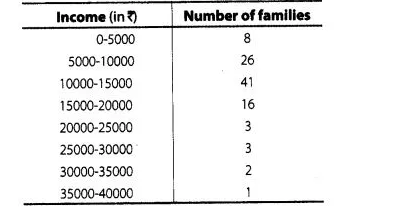# The monthly income of 100 families are given as below

Question:

The monthly income of 100 families are given as belowCaluculate the model income.

Solution:

In a given data, the highest frequency is 41, which lies in the interval 10000-15000.

Here, $l=10000, f_{m}=41, f_{1}=26, f_{2}=16$ and $h=5000$

$\therefore$ Mode $=l+\left(\frac{f_{m}-f_{1}}{2 f_{m}-t_{1}-f_{2}}\right) \times h$

$=10000+\left(\frac{41-26}{2 \times 41-26-16}\right) \times 5000$

$=10000+\left(\frac{15}{82-42}\right) \times 5000$

$=10000+\left(\frac{15}{40}\right) \times 5000$

$=10000+15 \times 125=10000+1875=₹ 11875$

Hence, the modal income is  ₹ 11875.